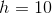# ACT Math : How to find the height of a 45/45/90 right isosceles triangle

## Example Questions

### Example Question #1 : How To Find The Height Of A 45/45/90 Right Isosceles Triangle

The area of an isosceles right triangle is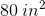.  What is its height that is correlative and perpendicular to a side that is not the hypotenuse?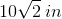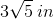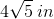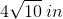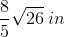Explanation:

Recall that an isosceles right triangle is a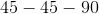triangle. That means that it looks like this: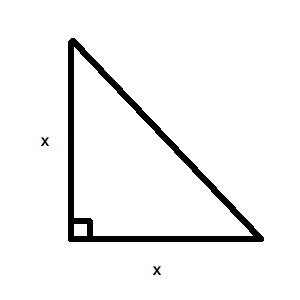This makes calculating the area very easy! Recall, the area of a triangle is defined as: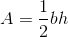However, since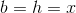for our triangle, we know: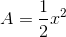Now, we know that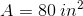. Therefore, we can write: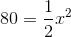Solving for, we get: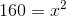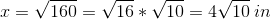This is the length of the height of the triangle for the side that is not the hypotenuse.

### Example Question #193 : Plane Geometry

What is the area of an isosceles right triangle that has an hypotenuse of length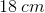?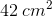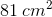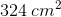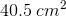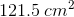Explanation:

Based on the information given, you know that your triangle looks as follows: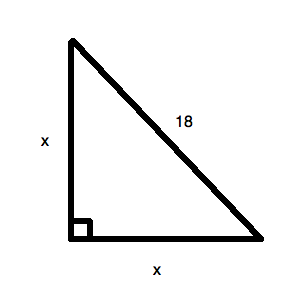This is atriangle. Recall your standardtriangle: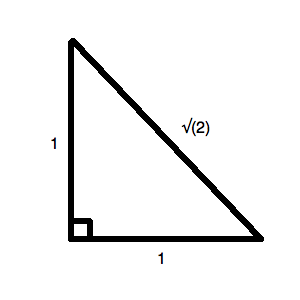You can set up the following ratio between these two figures: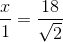Now, the area of the triangle will merely be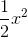(since both the base and the height are). For your data, this is: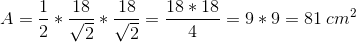### Example Question #194 : Plane Geometry

Find the height of an isoceles right triangle whose hypotenuse is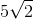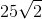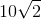Explanation:

To solve simply realize the hypotenuse of one of these triangles is of the form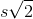where s is side length. Thus, our answer is.

### Example Question #195 : Plane Geometry

The area of an isosceles right triangle is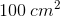. What is its height that is correlative and perpendicular to this triangle's hypotenuse?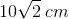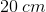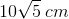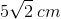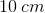Explanation:

Recall that an isosceles right triangle is atriangle. That means that it looks like this:This makes calculating the area very easy! Recall, the area of a triangle is defined as:However, sincefor our triangle, we know:Now, we know that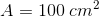. Therefore, we can write: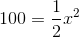Solving for, we get: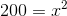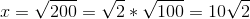However, be careful! Notice what the question asks: "What is its height that is correlative and perpendicular to this triangle's hypotenuse?" First, let's find the hypotenuse of the triangle. Recall your standardtriangle: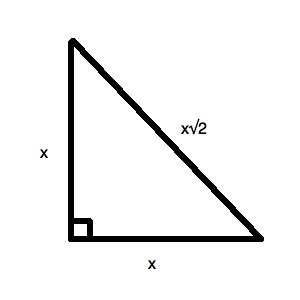Since one of your sides is, your hypotenuse is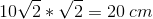.

Okay, what you are actually looking for isin the following figure: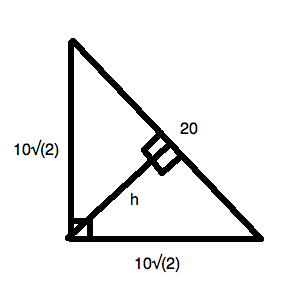Therefore, since you know the area, you can say: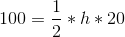Solving, you get: